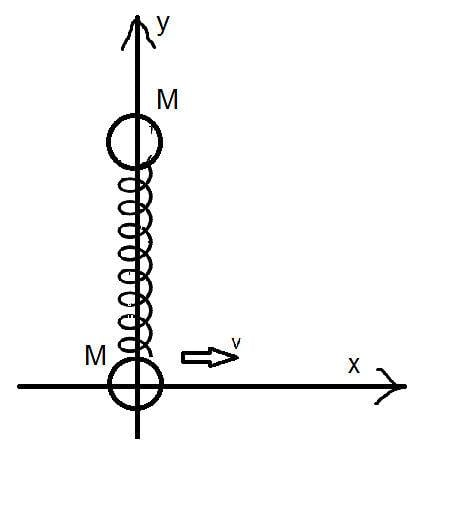# Instantaneous rotation centers

## Homework Statement

Is the conservation of angular momentum theorem right for instantaneous center rotation?
That: If all total momen of force is zero. The angular momentum will not change
If right, prove that.

## Homework Equations

All equations of rotational motion

## The Attempt at a Solution

I think it 's wrong because instantaneous center rotation always changes when object moves.

haruspex
Homework Helper
Gold Member

## Homework Statement

Is the conservation of angular momentum theorem right for instantaneous center rotation?
That: If all total momen of force is zero. The angular momentum will not change
If right, prove that.

## Homework Equations

All equations of rotational motion

## The Attempt at a Solution

I think it 's wrong because instantaneous center rotation always changes when object moves.
The safe points to use as axes for conservation of angular momentum are:
• Any point fixed in your inertial frame
• The mass centre of the rigid body
• The instantaneous centre of rotation of the rigid body
Interestingly, there are more. It can be shown that the set of points fixed relative to the body which also work form a circle passing through the mass centre and the instantaneous centre of rotation. However, that circle shifts over time.
Yes, the instantaneous centre of rotation might itself move. In that case, you can only use it for conservation over infinitesimal times.

•Hamal_Arietis
If the time is enough large, that 's right? Can you prove that by dynamical equations? I have to use this to solve another hard problem.
Two mass M are connected by spring has a stiffness k. Each body just moves on one-way. Initially, they are at ##(0,0)## and ##(0;y_0)## and the spring isnt deformed. So ##y_0=l_0## with ##l_0## is natural length of spring. Giving for body at (0;0) a velocity v. Find the equation of motion of system.Last edited:
haruspex
Homework Helper
Gold Member
If the time is enough large, that 's right?
Not sure what that was in response to. For moments about instantaneous centre of rotation I said "infinitesimal", i.e. arbitrarily small.
Anyway, for this question, conservation of angular momentum would not apply. Neither would linear momentum. The masses are constrained each to move in only one dimension, so there must be external forces.

External forces have directions to the instantanerious center rotation. So angular momentum with instantanerious center rotation will not change. If the problem was written in started is right.

haruspex
Homework Helper
Gold Member
External forces have directions to the instantanerious center rotation. So angular momentum with instantanerious center rotation will not change. If the problem was written in started is right.
It is true that you find where the external forces intersect, but, as you say, that point keeps moving, and we are not dealing with an instantaneous impulse here, so it won't help.
I don't understand why you want to bother with the instantaneous centre of rotation in this problem. How about a different conservation law?

•Hamal_Arietis
I think it very hard. Because all condition of problem suggests our to use started:
- The force of spring is the force inside.
- External forces have directions to the instantanerious center rotation.

haruspex